Function Repository Resource:

# BalancedTernary

Convert integers to and from balanced ternary

Contributed by: Ed Pegg Jr
 ResourceFunction["BalancedTernary"][n] toggles the n between integer and balanced ternary format.

## Details

ResourceFunction["BalancedTernary"] toggles between ordinary integer format and a format specific to the balanced ternary representation of an integer.
In balanced ternary,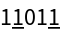represents 56.
+1×34-1×33+0×32+1×31-1×30 = 56.
{1,-1,0,1,-1}.3{4,3,2,1,0} = 56.
{1,-1,0,1,-1} is rewritten asfor balanced ternary.

## Examples

### Basic Examples (2)

Convert some integers to balanced ternary format:

 In:=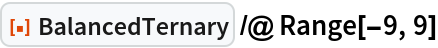Out=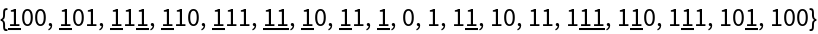Convert the balanced ternary numbers back into integers:

 In:=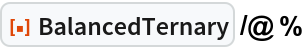Out=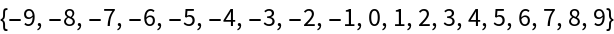### Scope (2)

Large numbers can be converted into balanced ternary:

 In:=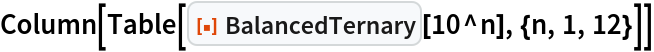Out=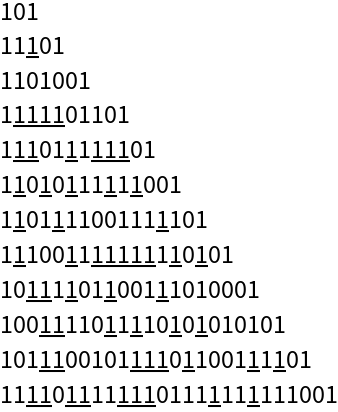Create a random 20-digit balanced ternary number:

 In:=Out=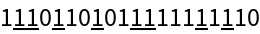Convert to an integer:

 In:=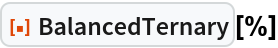Out=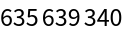### Properties and Relations (1)

Balanced ternary numbers are expressed in Row form:

 In:=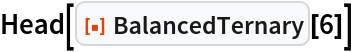Out=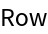### Neat Examples (1)

Using weights of size 1, 3, 9 and 27 and a 2-sided balance, measure weights 1 to 20:

 In:=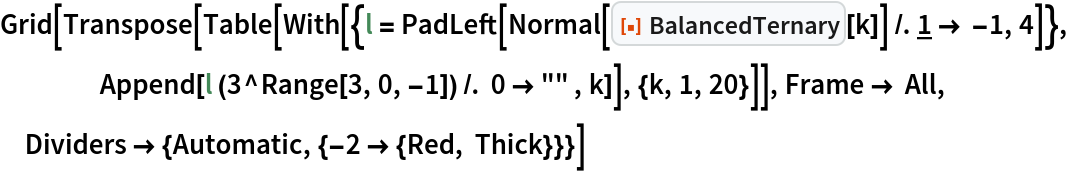Out=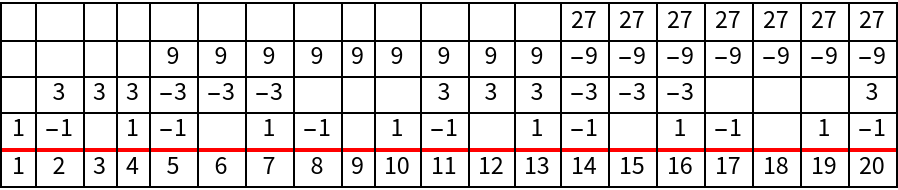## Version History

• 1.0.0 – 01 March 2021